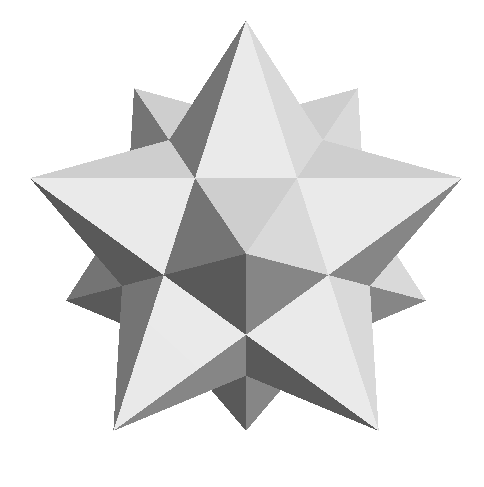• Polyhedron
• Videos
• Diagrams
• There are many KUSUDAMA Origami works shaped 'Stellation'.

But I decide the type of the polyhedron with a base shape consisting of the base region not a projection shape here.

Because the projection shape may not become the shape of Stellation.I summerized the 'regular polyhedron' and the 'half regular polyhedron' in a below list.

I separate it by color every regular polygon of each aspect and show the number of aspects

and the number of the side each(in ()).

If it is the origami unit such as the figure,

it becomes the number of the number = units of the side.These are all 18 kinds Kusudama examples of the regular polyhedron and the half regular polyhedron

with Bascetta Star modular.(because paper size is different, size is inconsistent...)